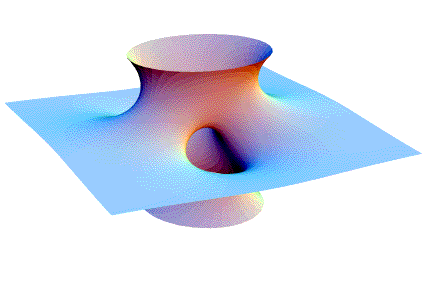A# minimal surfaceCosta's minimal surface.

A minimal surface is a surface that, bounded by a given closed curve or curves, has the smallest possible area. A minimal surface has a mean curvature of zero.

Finding and classifying minimal surfaces and proving that certain surfaces are minimal have been major mathematical problems for over 200 years. If the closed curve is planar then the solution is trivial; for example, the minimal surface bounded by a circle is just a disk. But the problem becomes much more difficult if the bounding curve is non-planar – in other words, is allowed to move up and down in the third dimension.

The first non-trivial examples of minimal surfaces, the catenoid and the helicoid, were discovered by the French geometer and engineer Jean Meusnier (1754–1793) in 1776, but there was then a gap of almost 60 years before the German Heinrich Scherk found some more. In 1873 the Belgian physicist Joseph Plateau carried out some experiments which led him to conjecture that soap bubbles and soap films always form minimal surfaces. Proving mathematically this was true became known as Plateau's problem.

Most minimal surfaces are extremely hard to construct and visualize, in part because the majority of them are self-intersecting. However, the development of high performance computer graphics has provided mathematicians with a powerful tool and the last couple of decades of seen a huge increase in the number of such surfaces that have been defined and investigated.